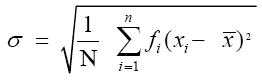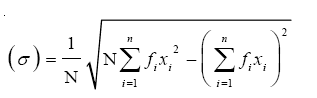# How To Find Standard Deviation Of Grouped Data

A standard deviation is a useful tool in investing and trading strategies. It is an important topic in statistics. Standard deviation calculates the dispersion of a dataset relative to its mean. If the data points are away from the mean, there is a higher deviation within the data set. Hence, the more spread out the data, the greater the standard deviation. Standard deviation cannot be negative. Mean is the average of a given set of observations. In this article, we will discuss how to find the standard deviation of grouped data.

## What is Standard Deviation?

Standard deviation calculates the dispersion of a dataset relative to its mean.

It is denoted by σ.

## How to calculate Standard Deviation of grouped data step by step?

Step 1. Make the frequency distribution table with 6 columns. Write class and frequency fi in first and second columns, respectively.

Step 2. Find N = ∑ni=1 fi.

Step 3. Find the midpoint of each class. This is denoted by xi. It is the average of the upper-class limit and the lower class limit. Write xi in the 3rd column.

Step 4. Find fixi (product of frequency and mid-point). Write those values in the 4th column.

Step 5. Find $\bar{x}$ using the formula (1/N) ∑ni=1 fixi.

Step 6. Find $(x_{i}-\bar{x})^{2}$ and enter in the 5th column.

Step 7. Find $f_{i}(x_{i}-\bar{x})^{2}$ and enter in the 6th column.

Step 8. Find $\sum f_{i}(x_{i}-\bar{x})^{2}$.

Step 9. Find standard deviation using the formula $\frac{1}{N}\sqrt{\sum f_{i}(x_{i}-\bar{x})^{2}}$.

### Formula

If the frequency distribution has n classes each class defined by its mid-point xi with frequency fi, the standard deviation can be calculated by the formulaWhere N = ∑ni=1 fi. $\bar{x}$ is the mean of the distribution.

Another formula for standard deviation is given byExample

The mean and S.D. of the marks of 200 candidates were found to be 40 and 15, respectively. Then, it was discovered that a score of 40 was wrongly read as 50. The correct mean and S.D., respectively are

(A) 14.98, 39.95

(B) 39.95, 14.98

(C) 39.95, 224.5

(D) None of these

Solution:

Given no. of candidates = 200

mean = 40

S.D = 15

Corrected ∑x = 40× 200 – 50+40 = 7990

Corrected $\bar{x}$ = 7990/200 = 39.95

Incorrect ∑x2 = n(σ2 + $\bar{x}^{2}$)

= 200(152+402)

= 200(225 + 1600)

= 365000

Correct ∑x2 = 365000-2500+1600

= 364100

σ = √((364100/200)-39.952)

= √(1820.5-1596.0025)

= √224.49

= 14.98

Hence option (B) is the answer.

Test your knowledge on How To Find Standard Deviation Of Grouped Data